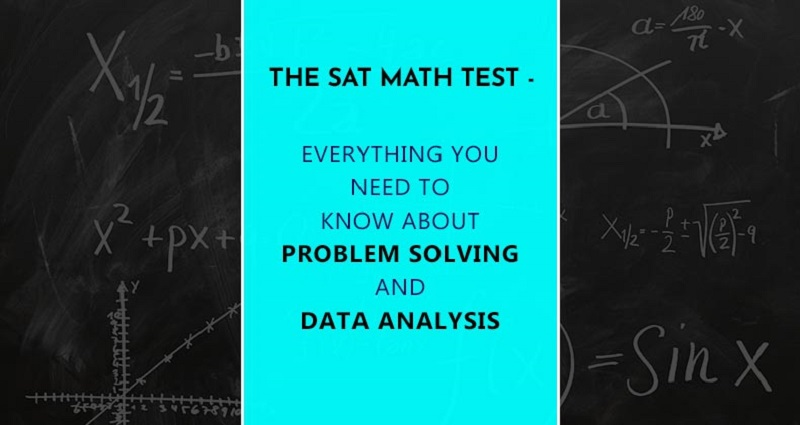X

# The SAT Math Test: Everything You Need To Know About Problem Solving And Data Analysis

Jun 27, 2018The SAT Math test falls into different groups called domains. Once of these groups is known as problem solving and data analysis.

Problem solving and data analysis questions may ask you to:

• Convert units,

• Form an appropriate equation from a given word problem,

• Comprehend the underlying meaning of different variables or numbers in an equation.

Problems belonging to this category may ask you to use your knowledge of rates, ratios and proportions for solutions. A few example problems that you’ll be asked to solve may include the likes of:

• Conversion of units (example, from meter/second to kilometer/hour).

• Choosing appropriate graphical representations for data sets.

• Interpretation of the slope and interception of the line.

• Computing and interpreting probability.

• Evaluating statistical claims, etc.Problem Solving And Data Analysis Syllabus

• Ratio, Proportion, Units and Percentages

• Charts, Graphs and Tables

• Data and Statistics

Sample Problems For Practice

Q1. The ratio of shirts to shorts to pairs of shoes in Kevin’s closet is 5 : 2 : 3. If Kevin owns 10 shirts, how many pairs of shoes does he have to give away so that he ends up having the same number of shorts as he does pairs of shoes?

A) 1

B) 2

C) 4

D) 5

Solution:

Since we know that Kevin has 10 shirts, and that 10 therefore corresponds to the number ‘5’ in the ratio, the actual number of shorts, shirts, etc., he owns is double the numbers in the ratio.

Therefore, he owns four shirts and six pairs of shoes. So he’ll have to give away 2 pairs of shoes so that he’ll have the same number of shoes as he does shorts.

Q2. There are 200,000 voters in district X, 60% of who voted in the 2008 state election. In 2010 state election, the number of voters in district X increased by 20% but if only 55% voted in this election, how many total votes were cast in the 2010 state election, assuming that no voter can cast more than one vote?

A) 12,000

B) 120,000

C) 132,000

D) 176,000

Solution:

Number of voters who voted in the 2008 election is equal to 200,000 x 60 = 120,000

In 2010, the number of overall voters increased by 20%, so 20% of 200,000 is 40,000 giving us 240,000 total voters.

55% of 240,000 gives us 132,000.

Q3. The regular rate for a massage at a spa clinic is $45 per session. The clinic currently offers a special package of$200 for 6 sessions. What percentage does a customer taking up this offer save for 6 sessions? Round your answer to the nearest whole percent?

A) 20%

B) 24%

C) 26%

D) 22%

Solution:

At the regular rate, 6 sessions will cost:

$45 x 6 =$270

The amount saved for 6 sessions when using the promo is:

$270 –$200 = \$70

That amount represents a percentage savings of:

70/270 * 100% = 25.9% = 26%

Q4. In a popular department store, a designer coat is discounted 20% off of the original price. After not selling for three months, the coat is further marked down another 20%. If the same coat sells online for 40% lower than the original department store price, how much percent less would somebody pay if they were to buy the coat directly online than if they were to buy the coat after it has been discounted twice at the department store?

A) 4%

B) 6.25%

C) 16%

D) 36%

Solution:

When you are not given a specific value for a percent problem, use 100 since it is easiest to increase or decrease in terms of %.

1st discount: 20% off of 100 = 80.

2nd discount: 20% off of 80 = 64.

Online, the coat sells for 40% off of the original department store price, which we assumed is 100.

Online discount: 40% of 100 = 60.

Percent difference: (64 – 60)/64 = 1/16 = 6.25%.

Q5. Sam takes an interstate road trip over the course of two days. If he covers 610 miles in nine hours the first day and 300 miles in four hours on the second day, what is the average speed per hour?

A) 55 miles per hour

B) 65 miles per hour

C) 70 miles per hour

D) 75 miles per hour

Solution:

To figure out the average speed of the entire trip, divide the total distance by the total number of hours.

D = rt, where D is total distance, r is rate, and t is time.

D = 910, r = ?, t = 9 + 4 = 13 hours.

910 = 13r, r = 70.

All the best for your SAT examination!

Article Posted in: Exam Preparation### MyPrivateTutor UAE

MyPrivateTutor is an online marketplace for all kinds of tutoring and learning services. We provide a platform to help learners find home tutors, online tutors, expert trainers,tutorial centers, training institutes and online tutoring schools. Learners can also find local classes, workshops, online courses and tutorials on a huge variety of topics. We operate in 12 countries worldwide and our community of learners, teachers and training businesses numbers over 200,000. We provide an opportunity for individual tutors and tutoring businesses to promote and grow their business.